# Circle simple

Circumference of a circle is 6.28. What is the area of the circle?

Correct result:

S =  3.1384

#### Solution: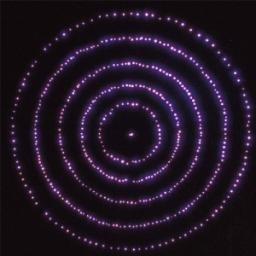We would be pleased if you find an error in the word problem, spelling mistakes, or inaccuracies and send it to us. Thank you!#### You need to know the following knowledge to solve this word math problem:

We encourage you to watch this tutorial video on this math problem:

## Next similar math problems:

• CircleHow big is area of circle if its circumference is 80.6 cm?
• Circle - simpleThe circumference of a circle is 198 mm. How long in mm is its diameter?
• Diameter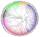The diameter of a circular plot is 14 dm. Find the circumference and area.
• Perimeter of squareThe square has a circumference 17cm. What is its area?
• Perimeter to areaCalculate the area of a circle with the perimeter 15 meters.
• Circle - easy 2The circle has a radius 6 cm. Calculate:
• Diameter to areaFind the area of a circle whose diameter is 26cm.
• ShapePlane shape has a maximum area 677 mm2. Calculate its perimeter if perimeter is the smallest possible.
• Square and circle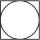Into square is inscribed circle with diameter 10 cm.What is difference between circumference square and circle?
• 22/7 circleCalculate approximately area of a circle with radius 20 cm. When calculating π use 22/7.
• Circle area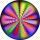Calculate the circle area with a radius of 1.2 m.
• Circle - simpleCalculate the area of a circle in dm2, if its circumference is 31.4 cm.
• Star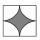Calculate the content of the shaded part of square with side 28 cm.
• Circle from string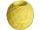Martin has a long 628 mm string . He makes circle from it. Calculate the radius of the circle.
• Calculate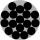Calculate the area of a circle whose radius is 5 dm.
• Rhombus HP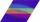Calculate area of the rhombus with height 24 dm and perimeter 12 dm.
• 6-gon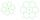Perimeter of regular hexagon is 113. Calculate its circumradius (radius of circumscribed circle).